# Economic dispatch in energy systems#

# install AMPL and solvers
%pip install -q amplpy pandas matplotlib numpy seaborn

SOLVER = "highs"

from amplpy import AMPL, ampl_notebook

ampl = ampl_notebook(
modules=["highs"],  # modules to install
)  # instantiate AMPL object and register magics

Using default Community Edition License for Colab. Get yours at: https://ampl.com/ce
Licensed to AMPL Community Edition License for the AMPL Model Colaboratory (https://colab.ampl.com).


# Chance-constrained economic energy dispatch problem#

In this notebook, we will explore the applications of chance constraints to an area where high-probability guarantees on the system’s functioning are required - the economic dispatch (ED) problem.

The problem considers the short-term determination of the optimal production of energy to meet all energy demands. Let $$V$$ denote a set of nodes, each of which is representing cities, industrial districts, power generators, or combinations of these. Each node $$i \in V$$ may have:

• a certain energy demand $$d_i \geq 0$$;

• a power generator whose energy production needs to be between $$p_i^{min}$$ and $$p_i^{max}$$ units of power. The cost of producing one unit of power at node $$i$$ is given by a variable cost $$c_i \geq 0$$. Importantly, not all the nodes have demand and generation, more specifically it is possible for a node to have only generation or only demand.

The goal is to determine for each node $$i \in V$$ the optimal production level $$p_i$$, such that

• the total energy demand is met

• no production limits are exceeded

• the total energy production costs are minimized.

If we fully control the energy production and the customer demand is known, can formulate the problem as the following MILO problem:

\begin{split} \begin{align} \begin{array}{llll} \min & \sum_{i \in V} c_i p_i\\ \text{s.t.} & \sum_{i \in V} p_i = \sum_{i \in V} d_i,\\ & p_{i}^{min} \leq p_{i} \leq p_{i}^{max} & \forall i \in V. \end{array} \end{align} \end{split}

Now, assume that we have built several offshore wind turbines. These wind turbines combined together produce a random non-negative amount of extra energy, denoted by $$\omega$$. For a fixed value of $$\omega$$, the optimization problem to be solved is thus to ‘fill in’ to the remaining energy demand not satisfied by wind power:

\begin{split} \begin{align} \begin{array}{llll} \min & \sum_{i \in V} c_i p_i\\ \text{s.t.} & \omega + \sum_{i \in V} p_i = \sum_{i \in V} d_i,\\ & p_{i}^{min} \leq p_{i} \leq p_{i}^{max} & \forall i \in V. \end{array} \end{align} \end{split}

The problem, however, is that $$\omega$$ is a random variable and is typically not fully known before the generation levels $$p_i$$ of conventional generators have to be set. Because of stochastic fluctuations in wind power generation, the ED problem is best modeled as a stochastic optimization problem. The intermittency of wind generation makes it almost impossible to perfectly balance supply and demand on a real-time basis, but in practice there is some tolerance for error, i.e., certain degree of mismatch between supply and demand can be easily adjusted for.

To formulate the problem under this assumption, let us denote by:

• $$\Delta \geq 0$$ the tolerance of the absolute power mismatch between supply and demand;

• $$\varepsilon \in [0,1]$$ is the risk level at which we are willing to accept for the supply to deviate from the demand more than $$\Delta$$;

• $$\omega$$ the non-negative random variable describing the total power production of offshore wind turbines.

In this setting, instead of requiring that the supply and demand are matched perfectly, we require that the absolute difference remains below power threshold $$\Delta$$ using the following chance constraint:

\begin{align} \mathbb{P} \Big ( \Big | \omega + \sum_{i \in V } p_i - \sum_{i \in V} d_i \Big | \leq \Delta \Big) \geq 1 - \varepsilon. \end{align}

To formulate the problem as an MILO, we can break up this absolute-value function including constraint into two individual chance constraints - note that in this way we relax the constraint because requiring that two one-sided constraints hold with probability $$1 - \varepsilon$$ each is not the same as requiring that together they hold with probability $$1 - \varepsilon$$, but in practice we can fine-tune the $$\varepsilon$$ to adapt for this change.

Such breaking up leads us to the following optimization problem with chance constraints:

\begin{split} \begin{align} \begin{array}{llll} \min & \sum_{i \in V } c_i p_i\\ \text{s.t.} & \mathbb{P}(\omega + \sum_{i \in V } p_i - \sum_{i \in V} d_i \leq \Delta) \geq 1 - \varepsilon\\ & \mathbb{P}(\omega + \sum_{i \in V } p_i - \sum_{i \in V} d_i \geq -\Delta) \geq 1 - \varepsilon\\ & p_{i}^{min } \leq p_{i} \leq p_{i}^{max } & \forall i \in V. \end{array} \end{align} \end{split}

In this notebook, we will solve this problem using the SAA approach to chance constraints, with the wind power production using modeled with historical data of 500 outcomes of the total wind production.

## Data import#

We first import the necessary packages and define a function that reads all the necessary node and wind production random sample data.

import numpy as np
import pandas as pd
import matplotlib.pyplot as plt
import seaborn as sns

def read_economic_dispatch_data():
# Read local nodes.csv if exists
base_url = "https://raw.githubusercontent.com/mobook/MO-book/main/notebooks/09/"
nodes_df = pd.read_csv(base_url + "nodes.csv", index_col=0)[
["node_id", "d", "p_min", "p_max", "c_var"]
]

nodes = nodes_df.set_index("node_id").T.to_dict()
wind_production_samples = list(wind_production_samples_df.to_dict().values())
wind_production_samples = [sum(d.values()) for d in wind_production_samples]

return nodes, wind_production_samples



The wind production samples can be accessed through the wind_production_samples variable - a list of 500 equiprobable outcomes for the wind generation.

wind_production_samples  # fifth outcome

196.94632359541376


Let us take a look into the wind production data, including a Kernel Density Estimate. We see that is has two modes. This paper explains what one could do with this.

sns.set_style("darkgrid")
sns.histplot(
wind_production_samples, kde=True, stat="density", kde_kws=dict(cut=1)
).set_title("wind production")
plt.show()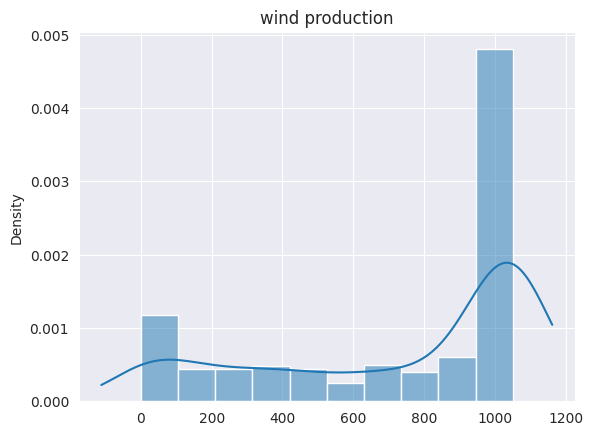The nodes dictionary contains for every $$i \in V$$ information about $$p_i^{min}$$, $$p_i^{max}$$, $$c_i$$, $$d_i$$. In our dataset, there is a clear separation of the nodes into nodes that only consume power, and nodes that only produce power, which can be seen by inspecting the node properties.

nodes  # first node properties

{'d': 44.23034433319671, 'p_min': 0.0, 'p_max': 0.0, 'c_var': 0.0}


Let us now provide some locations to our producers and consumers and visualize the data, using bubbles proportional to the size of demand (for consumers) and maximum generation capacity (for producers).

df_nodes = pd.DataFrame.from_dict(nodes, orient="index")
df_nodes["type"] = "consumer"
df_nodes.loc[df_nodes.p_max > 0, "type"] = "producer"
np.random.seed(2023)
df_nodes["x"] = np.random.randint(0, 100, len(nodes))
df_nodes["y"] = np.random.randint(0, 100, len(nodes))
df_nodes["size"] = df_nodes[["d", "p_max"]].max(axis=1)

def ShowInstance(df_nodes):
# Define the size of the figure
fig, ax = plt.subplots(figsize=(8, 6))

# Set the scales of the x and y axes
ax.set_xlim([min(df_nodes["x"]) - 10, max(df_nodes["x"]) + 10])
ax.set_ylim([min(df_nodes["y"]) - 10, max(df_nodes["y"]) + 10])

# Create a scatter plot with bubbles proportional to size
# ax.scatter(df_nodes['x'], df_nodes['y'], s=df_nodes['size']*1)
for (category, group), z, color in zip(
df_nodes.groupby("type"), [2, 1], ["red", "green"]
):
ax.scatter(
group.x,
group.y,
s=group["size"] * 1,
label=category,
alpha=0.2,
zorder=z,
color=color,
)
if "sol" in group:
ax.scatter(
group.x,
group.y,
s=group["sol"] * 1,
label=None,
alpha=1,
zorder=z,
color=color,
)

plt.legend()
# Show the chart
plt.show()

ShowInstance(df_nodes)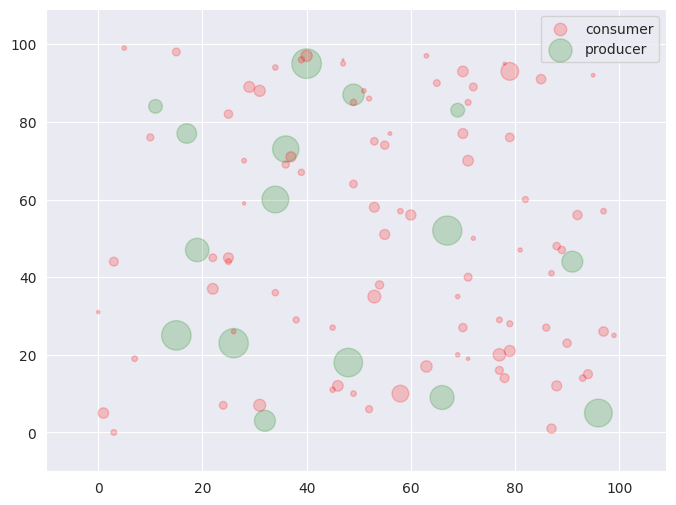## MILO reformulation for the chance-constrained ED problem#

Since we have a discrete set of $$N$$ possible wind production outcomes, we can reformulate the chance-constrained ED problem as a mixed-integer linear optimization problem. More specifically, we introduce a binary variable $$u_j$$ for each sample $$j$$ of the wind production, which, thanks to the big-$$M$$ technique, determines whether the constraint related to the $$j$$-th sample is allowed to be violated or not, and add one constraint to ensure that the total probability that the constraint is violated is at most $$\varepsilon$$, i.e.,

$\frac{1}{N} \sum_{j=1}^{N} u_j \leq \varepsilon.$

The resulting MILO is

\begin{split} \begin{align} \begin{array}{llll} \min & \sum_{i \in V} c_i(p_i)\\ \text{s.t.} & \omega_j + \sum_{i \in V} p_i - \sum_{i \in V} d_i \leq \Delta + u_jM_j & \forall j = 1, \dots, N\\ & \omega_j + \sum_{i \in V} p_i - \sum_{i \in V} d_i \geq -\Delta - u_jM_j & \forall j = 1, \dots, N\\ & \sum_{j=1}^{N}u_j \leq \varepsilon N \\ & p_{i}^{min } \leq p_{i} \leq p_{i}^{max } & \forall i \in V, \\ & u_j \in \{0, 1\} & \forall j = 1, ..., N, \end{array} \end{align} \end{split}

For each sample, the supply and demand constraints are deactivated when $$u_j =1$$ and $$u_j=0$$ otherwise. Note that we only use one single $$u_j$$ variable for each constraint. Indeed, having two separate $$u^{(1)}_j$$ and $$u^{(2)}_j$$ will yield the same objective value, but the model would be incorrect with respect to the violation of the supply and demand constraints introduced earlier.

The constants $$M_j$$’s here should be selected based on the data: one reasonable choice for $$M_j$$ that will certainly work out is to take it equal to the left-hand side minus $$\Delta$$ while replacing $$p_i$$ for $$p_i^{max}$$.

We now define the Python function which implements this model. Note that the model is formulated with $$\varepsilon$$ and $$\Delta$$ as mutable model parameters, so that we can repeatedly solve the same model instance, upon modifying the parameters. In turn, this requires the $$M_j$$ constants to be expressions.

%%writefile economic_dispatch.mod

param N; # number of samples
param samples{1..N};

param eps;
param Delta;

set V;
param c{V};
param d{V};
param p_min{V};
param p_max{V};

param sum_pmax = sum{i in V} p_max[i];
param sum_d = sum{i in V} d[i];

param M{j in 1..N} = samples[j] + sum_pmax + sum_d - Delta;

var p{i in V} >= p_min[i], <= p_max[i];
var u{i in 1..N} binary;

minimize my_objective: sum{i in V}(c[i] * p[i]);

s.t. supply_demand_leq {j in 1..N}:
samples[j] + sum{i in V} p[i] - sum{i in V} d[i] <= Delta + M[j] * u[j];
s.t. supply_demand_geq {j in 1..N}:
samples[j] + sum{i in V} p[i] - sum{i in V} d[i] >= - Delta - M[j] * u[j];
s.t. success_probability: sum{j in 1..N} u[j] <= eps * N;

Overwriting economic_dispatch.mod

def economic_dispatch(nodes_df, samples, eps, Delta):
# Create AMPL instance and load the model
ampl = AMPL()

ampl.set["V"] = nodes_df["node_id"]
ampl.param["c"] = nodes_df["c_var"]
ampl.param["p_min"] = nodes_df["p_min"]
ampl.param["p_max"] = nodes_df["p_max"]
ampl.param["d"] = nodes_df["d"]

ampl.param["N"] = len(samples)
ampl.param["samples"] = samples

ampl.param["eps"] = eps
ampl.param["Delta"] = Delta

# Set solver
ampl.option["solver"] = SOLVER

return ampl


For demonstration purposes, we now solve the model for the provided instance and wind production outcomes and report the optimal objective value you obtain for $$\varepsilon = 0.02$$ and $$\Delta=1000$$.

# Data
eps = 0.20
Delta = 1000
N = 500

base_url = "https://raw.githubusercontent.com/mobook/MO-book/main/notebooks/09/"
# Dataframe with node information
nodes_df = pd.read_csv(base_url + "nodes.csv", index_col=0)[
["node_id", "d", "p_min", "p_max", "c_var"]
]

# Solve model and report the solution
model = economic_dispatch(nodes_df, wind_production_samples, eps, Delta)
model.solve()

sum_production = model.get_value("sum{i in V} p[i]")
sum_demand = sum(nodes_df["d"])
print(f"Total energy demand: {sum_demand:.3f}")
print(f"Total optimal energy production: {sum_production:.3f}")
print(f"Total energy production cost: {model.obj['my_objective'].value():.3f}")

HiGHS 1.5.3: HiGHS 1.5.3: optimal solution; objective 7850.60066
12071 simplex iterations
1 branching nodes

Total energy demand: 3007.112
Total optimal energy production: 1733.393
Total energy production cost: 7850.601


# Visualizing and understanding the solution#

df_nodes["sol"] = model.var["p"].get_values().toPandas()
ShowInstance(df_nodes)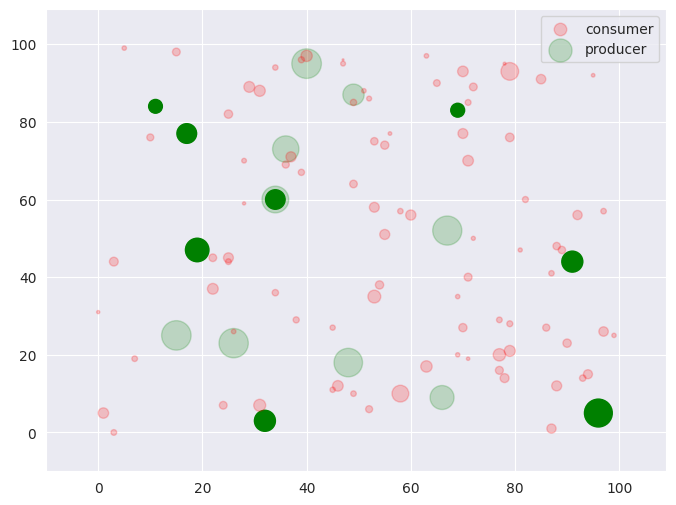## Sensitivity analysis#

Next, we will study the sensitivity of the optimal solution and value to the different risk guarantee levels - this helps the decision maker find a level that offers the best risk-reward tradeoff. To this end, we solve the same MILO varying the values first of $$\varepsilon \in [0, 1]$$ (for fixed $$\Delta=1000$$) and then of $$\Delta \in [0, 2000]$$ (for fixed $$\varepsilon = 0.02$$).

fixed_Delta = 1000

feas_eps = []
feas_objs = []

eps = 0
model = economic_dispatch(nodes_df, wind_production_samples, eps, fixed_Delta)

for eps in np.linspace(0, 1, num=20):
model.param["eps"] = eps
model.solve()

if model.get_value("solve_result") == "solved":
feas_eps.append(eps)
feas_objs.append(model.obj["my_objective"].value())

plt.plot(feas_eps, feas_objs, marker="o", linestyle="--")
plt.xlabel("$\epsilon$")
plt.ylabel("objective value")
plt.show()

HiGHS 1.5.3: HiGHS 1.5.3: optimal solution; objective 13051.25426
1 simplex iterations
0 barrier iterations

HiGHS 1.5.3: HiGHS 1.5.3: optimal solution; objective 13027.32641
2028 simplex iterations
1 branching nodes

HiGHS 1.5.3: HiGHS 1.5.3: optimal solution; objective 11790.73881
7076 simplex iterations
1 branching nodes

HiGHS 1.5.3: HiGHS 1.5.3: optimal solution; objective 9577.271586
9076 simplex iterations
1 branching nodes

HiGHS 1.5.3: HiGHS 1.5.3: optimal solution; objective 7461.511327
15901 simplex iterations
8 branching nodes

HiGHS 1.5.3: HiGHS 1.5.3: optimal solution; objective 5270.132613
11448 simplex iterations
1 branching nodes

HiGHS 1.5.3: HiGHS 1.5.3: optimal solution; objective 2781.668242
2905 simplex iterations
1 branching nodes

HiGHS 1.5.3: HiGHS 1.5.3: optimal solution; objective 383.7998865
894 simplex iterations
1 branching nodes

HiGHS 1.5.3: HiGHS 1.5.3: optimal solution; objective 0
0 simplex iterations
1 branching nodes

HiGHS 1.5.3: HiGHS 1.5.3: optimal solution; objective 0
0 simplex iterations
0 branching nodes

HiGHS 1.5.3: HiGHS 1.5.3: optimal solution; objective 0
0 simplex iterations
0 branching nodes

HiGHS 1.5.3: HiGHS 1.5.3: optimal solution; objective 0
0 simplex iterations
0 branching nodes

HiGHS 1.5.3: HiGHS 1.5.3: optimal solution; objective 0
0 simplex iterations
0 branching nodes

HiGHS 1.5.3: HiGHS 1.5.3: optimal solution; objective 0
0 simplex iterations
0 branching nodes

HiGHS 1.5.3: HiGHS 1.5.3: optimal solution; objective 0
0 simplex iterations
0 branching nodes

HiGHS 1.5.3: HiGHS 1.5.3: optimal solution; objective 0
0 simplex iterations
0 branching nodes

HiGHS 1.5.3: HiGHS 1.5.3: optimal solution; objective 0
0 simplex iterations
0 branching nodes

HiGHS 1.5.3: HiGHS 1.5.3: optimal solution; objective 0
0 simplex iterations
0 branching nodes

HiGHS 1.5.3: HiGHS 1.5.3: optimal solution; objective 0
0 simplex iterations
0 branching nodes

HiGHS 1.5.3: HiGHS 1.5.3: optimal solution; objective 0
0 simplex iterations
0 branching nodes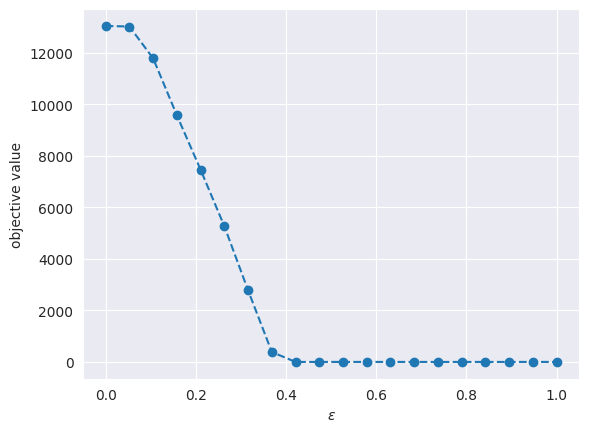fixed_eps = 0.02

feas_Deltas = []
feas_objs = []

Delta = 0
model = economic_dispatch(nodes_df, wind_production_samples, fixed_eps, Delta)

for Delta in np.linspace(0, 2000, num=20):
model.param["Delta"] = Delta
model.solve()

if model.get_value("solve_result") == "solved":
feas_Deltas.append(Delta)
feas_objs.append(model.obj["my_objective"].value())

plt.plot(feas_Deltas, feas_objs, marker="o", linestyle="--")
plt.xlabel("$\Delta$")
plt.ylabel("objective value")
plt.show()

HiGHS 1.5.3: HiGHS 1.5.3: infeasible problem
120 simplex iterations
1 branching nodes

HiGHS 1.5.3: HiGHS 1.5.3: infeasible problem
133 simplex iterations
1 branching nodes

HiGHS 1.5.3: HiGHS 1.5.3: infeasible problem
150 simplex iterations
1 branching nodes

HiGHS 1.5.3: HiGHS 1.5.3: infeasible problem
190 simplex iterations
1 branching nodes

HiGHS 1.5.3: HiGHS 1.5.3: infeasible problem
423 simplex iterations
1 branching nodes

HiGHS 1.5.3: HiGHS 1.5.3: optimal solution; objective 25097.93105
444 simplex iterations
1 branching nodes

HiGHS 1.5.3: HiGHS 1.5.3: optimal solution; objective 22150.56263
814 simplex iterations
1 branching nodes

HiGHS 1.5.3: HiGHS 1.5.3: optimal solution; objective 19203.19421
908 simplex iterations
1 branching nodes

HiGHS 1.5.3: HiGHS 1.5.3: optimal solution; objective 16255.82579
2085 simplex iterations
1 branching nodes

HiGHS 1.5.3: HiGHS 1.5.3: optimal solution; objective 14051.25426
408 simplex iterations
1 branching nodes

HiGHS 1.5.3: HiGHS 1.5.3: optimal solution; objective 12051.25426
348 simplex iterations
1 branching nodes

HiGHS 1.5.3: HiGHS 1.5.3: optimal solution; objective 10051.25426
330 simplex iterations
1 branching nodes

HiGHS 1.5.3: HiGHS 1.5.3: optimal solution; objective 8051.254261
317 simplex iterations
1 branching nodes

HiGHS 1.5.3: HiGHS 1.5.3: optimal solution; objective 6051.254261
543 simplex iterations
1 branching nodes

HiGHS 1.5.3: HiGHS 1.5.3: optimal solution; objective 4053.447124
277 simplex iterations
1 branching nodes

HiGHS 1.5.3: HiGHS 1.5.3: optimal solution; objective 2158.710282
294 simplex iterations
1 branching nodes

HiGHS 1.5.3: HiGHS 1.5.3: optimal solution; objective 263.9734402
146 simplex iterations
1 branching nodes

HiGHS 1.5.3: HiGHS 1.5.3: optimal solution; objective 0
0 simplex iterations
1 branching nodes

HiGHS 1.5.3: HiGHS 1.5.3: optimal solution; objective 0
0 simplex iterations
0 branching nodes

HiGHS 1.5.3: HiGHS 1.5.3: optimal solution; objective 0
0 simplex iterations
0 branching nodes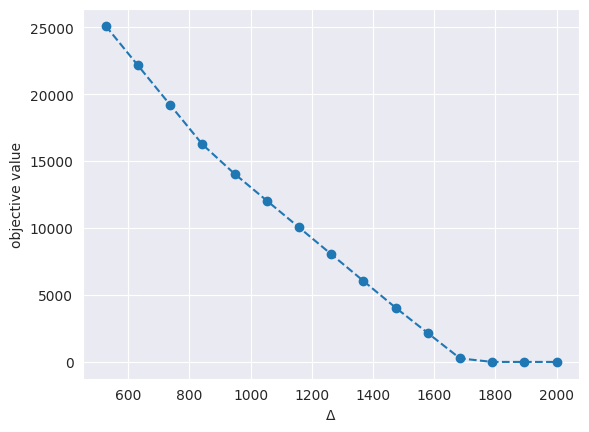Based on the above plots, we can make the following observations:

• Smaller values $$\varepsilon$$ and $$\Delta$$ lead to more infeasibilities, which is to be expected. Smaller $$\varepsilon$$ allow for less constraint violations/relaxations, whereas smaller Delta make constraints tighter (and thus easier to violate).

• The reason why the plot becomes flat for high $$\varepsilon$$ and $$\Delta$$ values is because production is no longer needed. For instance, a high $$\varepsilon$$ means we can ignore the $$N\varepsilon$$ worst-case sample scenarios, whereas higher $$\Delta$$ means that we do not need to produce to match demand.